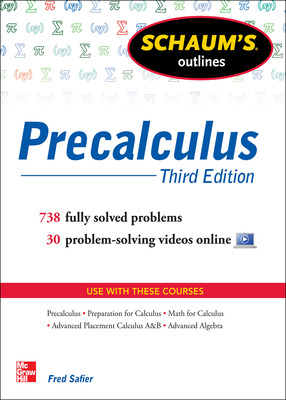# Schaum's Outline of Precalculus, 3rd Edition

3rd Edition
By Fred Safier
ISBN10: 0071795596
ISBN13: 9780071795593
Copyright: 2013

## Purchase Options

Students, we’re committed to providing you with high-value course solutions backed by great service and a team that cares about your success. See tabs below to explore options and pricing. Don't forget, we accept financial aid and scholarship funds in the form of credit or debit cards.

### Product

The estimated amount of time this product will be on the market is based on a number of factors, including faculty input to instructional design and the prior revision cycle and updates to academic research-which typically results in a revision cycle ranging from every two to four years for this product. Pricing subject to change at any time.

### Program Details

• Polynomials Exponents. • Rational and Radical Expressions• Linear and Non-Linear Equations • Linear and Non-Linear Inequalities • Absolute Value in Equations and Inequalities.• Analytic Geometry • Functions • Linear Functions • Transformations and Graphs• Quadratic Functions • Algebra of Functions • Polynomial Functions • Rational Functions • Algebraic Functions; Variations • Exponential Functions • Logarithmic Functions • Exponential and Logarithmic Equations • Trigonometric Functions • Graphs of Trigonometric Functions • Angles • Trigonometric Identities and Equations • Sum, Difference, Multiple, and • Half-Angle Formulas • Inverse Trigonometric Functions. • Triangles • Vectors • Polar Coordinates; Parametric Equations • Trigonometric Form of Complex Numbers • Systems of Linear Equations • Gaussian and Gauss-Jordan Elimination • Partial Fraction • Decomposition • Non-Linear Systems of Equations • Introduction to Matrix Algebra • Matrix Multiplication and Inverses • Determinants and Cramer's Rule. • Loci; Parabolas • Ellipses and Hyperbolas • Rotation of Axes • Conic Sections • Sequences and Series • The Principle of Mathematical Induction • Special Sequences and Series• The Binomial Theorem